All Modules View all topics Close topics
Rettifiche di giorno di bilanciamento nel giornale generale e nel registro

# Report

• Study Reminders

We'll email you at these times to remind you to study

You can set up to 7 reminders per week

#### You're all set

We'll email you at these times to remind you to study

Monday

Tuesday

Wednesday

Thursday

Friday

Saturday

Sunday

• Note di Apprendimento
• Revisione degli argomenti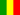Gabriel O. 1 0 Straight - line method assumes equal amounts of depreciation over an asset's useful life and this translates to equal depreciation expense amounts every period . The formula for calculating straight- line method is : COST - SAVING VALUE divided by useful life . Where : cost=purchase price useful life= estimated amount of time that the asset will be used by the company.this is sometimes called service life . salvage value= estimated amount the asset can be sold for at the end of its useful life . some times called residual value .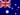Wendy C. 1 0 In reporting there are often a variety of classifications that are relevant for an entry.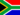Samuel F. 1 0 With regard to depreciation, what does the term mid-month convention mean?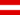Harrison A. 0 0 Straight - line method assumes equal amounts of depreciation over an asset's useful life and this translates to equal depreciation expense amounts every period . The formula for calculating straight- line method is : COST - SAVING VALUE divided by useful life . Where : cost=purchase price useful life= estimated amount of time that the asset will be used by the company.this is sometimes called service life . salvage value= estimated amount the asset can be sold for at the end of its useful life . some times called residual value .Harrison A. 1 0 which ways are depreciation calculated ?Zachary B. 0 0 What is reporting?Diamond T. 1 0 With regard to depreciation, what does the term mid-month convention mean?Douglas R. 1 0 Reporting vehicle depreciation using the horizontal line method.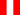Erick V. 0 0 En la declaración de pérdidas y ganancias depreciación del vehículo pueden clasificarse en venta, entrega o gasto vehículo tambien habría que considerar el costo de mantenimiento frente a la adqusicion de uno nuevo y su rentabilidad empresa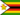Pride C. 0 0 Straight - line method assumes equal amounts of depreciation over an asset's useful life and this translates to equal depreciation expense amounts every period . The formula for calculating straight- line method is : COST - SAVING VALUE divided by useful life . Where : cost=purchase price useful life= estimated amount of time that the asset will be used by the company.this is sometimes called service life . salvage value= estimated amount the asset can be sold for at the end of its useful life . some times called residual value .
• Text Version